# 6.1. Extracting times series to build a functional connectome#

## 6.1.1. Time-series from a brain parcellation or “MaxProb” atlas#

### 6.1.1.1. Brain parcellations#

Regions used to extract the signal can be defined by a “hard” parcellation. For instance, the `nilearn.datasets` has functions to download atlases forming reference parcellation, e.g., `fetch_atlas_craddock_2012`, `fetch_atlas_harvard_oxford`, `fetch_atlas_yeo_2011`.

For instance to retrieve the Harvard-Oxford cortical parcellation, sampled at 2mm, and with a threshold of a probability of 0.25:

```from nilearn import datasets
dataset = datasets.fetch_atlas_harvard_oxford('cort-maxprob-thr25-2mm')
atlas_filename = dataset.maps
labels = dataset.labels
```

Plotting can then be done as:

```from nilearn import plotting
plotting.plot_roi(atlas_filename)
```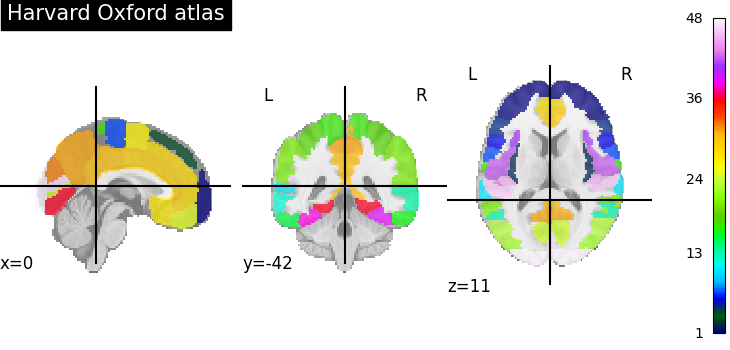### 6.1.1.2. Extracting signals on a parcellation#

To extract signal on the parcellation, the easiest option is to use the `NiftiLabelsMasker`. As any “maskers” in nilearn, it is a processing object that is created by specifying all the important parameters, but not the data:

```from nilearn.maskers import NiftiLabelsMasker
```

The Nifti data can then be turned to time-series by calling the `NiftiLabelsMasker.fit_transform` method, that takes either filenames or NiftiImage objects:

```time_series = masker.fit_transform(frmi_files,
confounds=confounds_dataframe)
```

Note that confound signals can be specified in the call. Indeed, to obtain time series that capture well the functional interactions between regions, regressing out noise sources is very important [Varoquaux & Craddock 2013]. For data processed by fMRIPrep, `load_confounds` and `load_confounds_strategy` can help you retrieve confound variables. `load_confounds_strategy` selects confounds based on past literature with limited parameters for customisation. For more freedoms of confounds selection, `load_confounds` groups confound variables as sets of noise components and one can fine tune each of the parameters.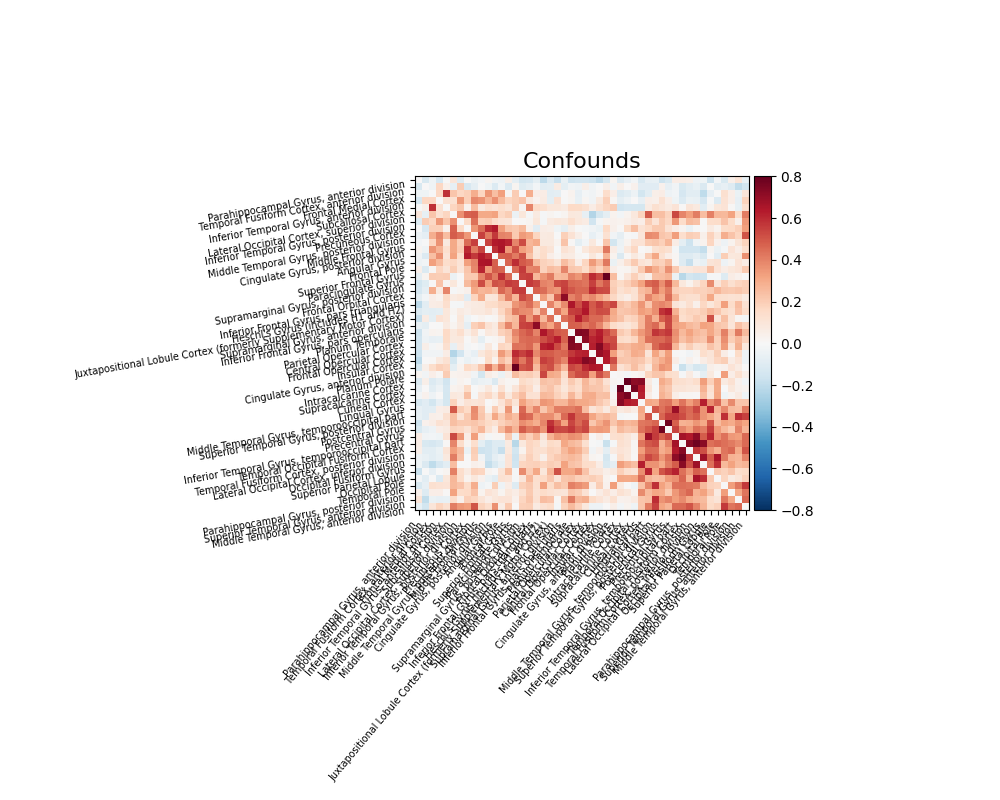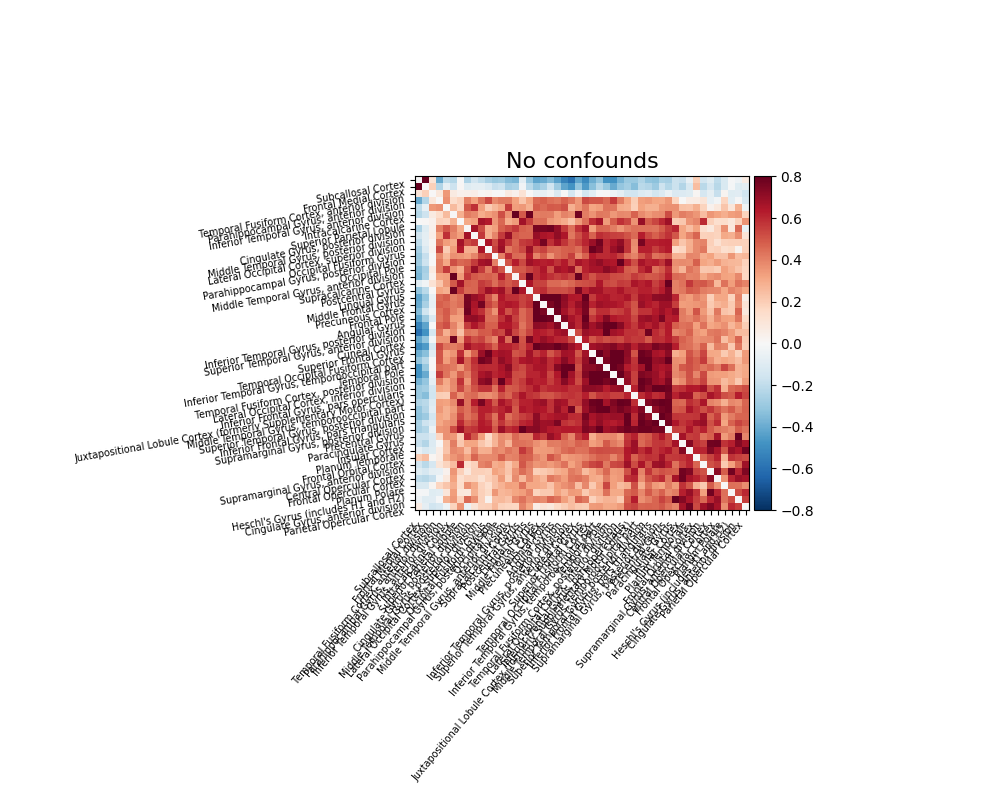## 6.1.2. Time-series from a probabilistic atlas#

### 6.1.2.1. Probabilistic atlases#

The definition of regions as by a continuous probability map captures better our imperfect knowledge of boundaries in brain images (notably because of inter-subject registration errors). One example of such an atlas well suited to resting-state or naturalistic-stimuli data analysis is the MSDL atlas (`nilearn.datasets.fetch_atlas_msdl`).

Probabilistic atlases are represented as a set of continuous maps, in a 4D nifti image. Visualization the atlas thus requires to visualize each of these maps, which requires accessing them with `nilearn.image.index_img` (see the corresponding example).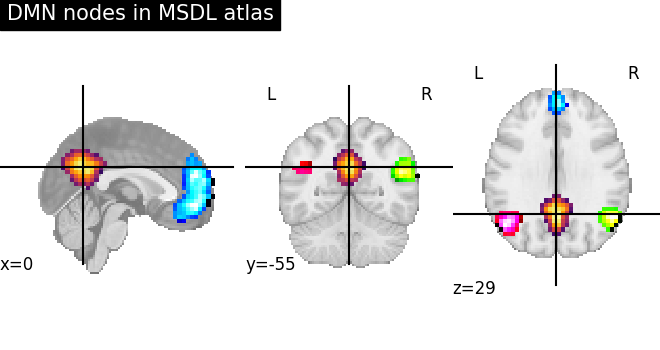### 6.1.2.2. Extracting signals from a probabilistic atlas#

As with extraction of signals on a parcellation, extracting signals from a probabilistic atlas can be done with a “masker” object: the `NiftiMapsMasker`. It is created by specifying the important parameters, in particular the atlas:

```from nilearn.maskers import NiftiMapsMasker
```

The fit_transform method turns filenames or NiftiImage objects to time series:

```time_series = masker.fit_transform(frmi_files, confounds=csv_file)
```

The procedure is the same as with brain parcellations but using the `NiftiMapsMasker`, and the same considerations on using confounds regressors apply.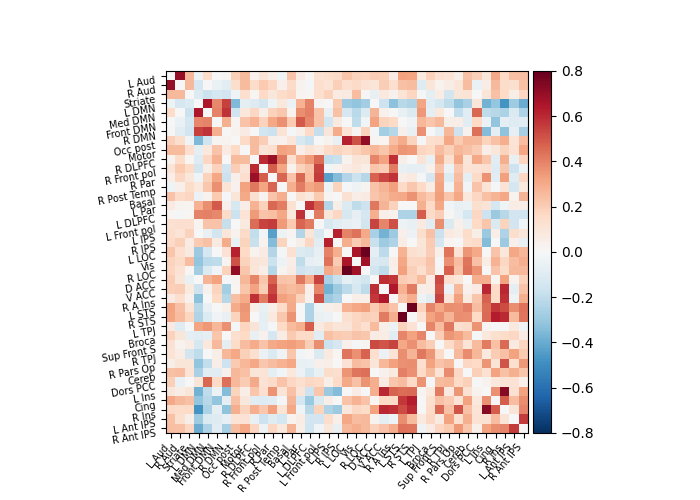## 6.1.3. A functional connectome: a graph of interactions#

A square matrix, such as a correlation matrix, can also be seen as a “graph”: a set of “nodes”, connected by “edges”. When these nodes are brain regions, and the edges capture interactions between them, this graph is a “functional connectome”.

We can display it with the `nilearn.plotting.plot_connectome` function that take the matrix, and coordinates of the nodes in MNI space. In the case of the MSDL atlas (`nilearn.datasets.fetch_atlas_msdl`), the CSV file readily comes with MNI coordinates for each region (see for instance example: Extracting signals of a probabilistic atlas of functional regions).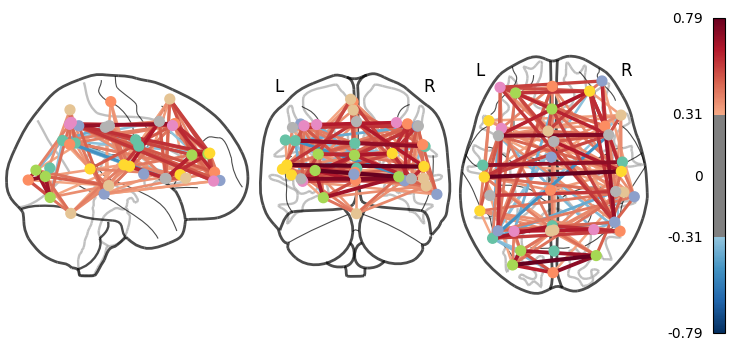As you can see, the correlation matrix gives a very “full” graph: every node is connected to every other one. This is because it also captures indirect connections. In the next section we will see how to focus on direct connections only.

## 6.1.4. A functional connectome: extracting coordinates of regions#

For atlases without readily available label coordinates, center coordinates can be computed for each region on hard parcellation or probabilistic atlases.